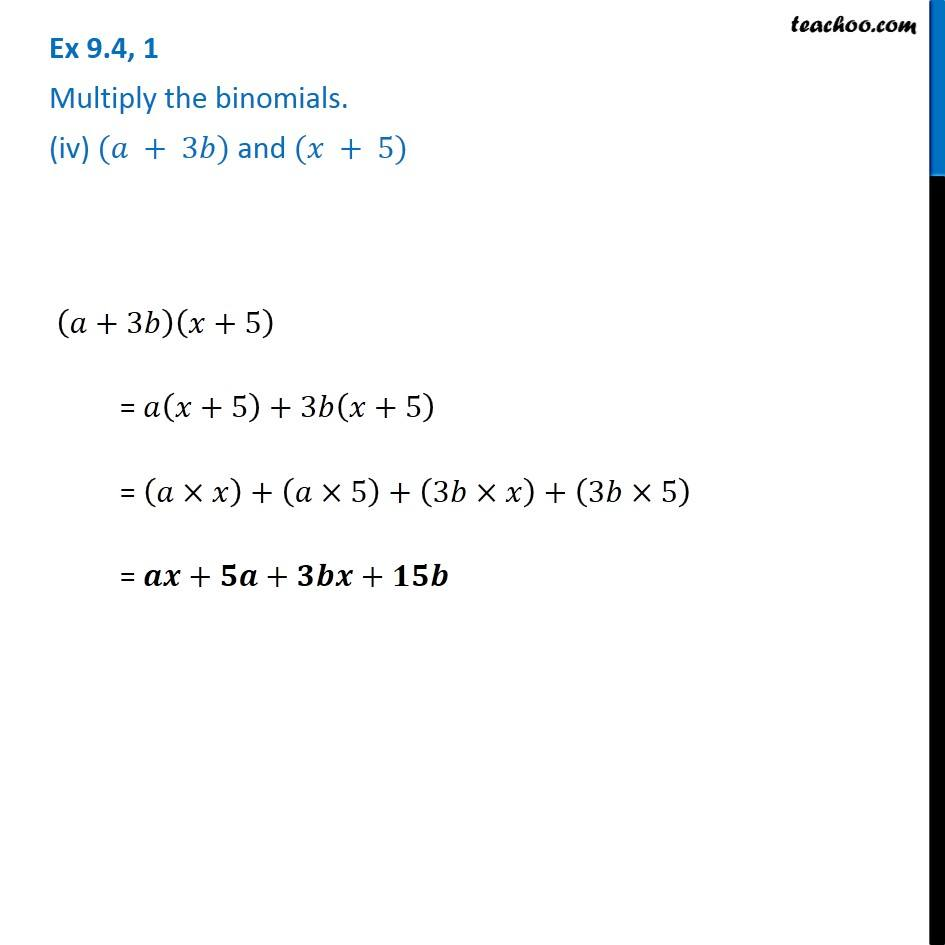1. Chapter 9 Class 8 Algebraic Expressions and Identities
2. Serial order wise
3. Ex 9.4

Transcript

Ex 9.4, 1 Multiply the binomials. (iv) (𝑎 + 3𝑏) and (𝑥 + 5) (𝑎+3𝑏)(𝑥+5) = 𝑎(𝑥+5)+3𝑏(𝑥+5) = (𝑎×𝑥)+(𝑎×5)+(3𝑏×𝑥)+(3𝑏×5) = 𝒂𝒙+𝟓𝒂+𝟑𝒃𝒙+𝟏𝟓𝒃